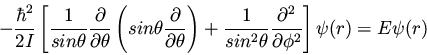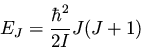Next: The Hydrogen Atom Up: Some Analytically Soluble Problems Previous: The Harmonic Oscillator

## The Rigid Rotor

The rigid rotor is a simple model of a rotating diatomic molecule. We consider the diatomic to consist of two point masses at a fixed internuclear distance. We then reduce the model to a one-dimensional system by considering the rigid rotor to have one mass fixed at the origin, which is orbited by the reduced mass, at a distance r. The Schrödinger equation is (cf. McQuarrie , section 6.4 for a clear explanation)(123)

After a little effort, the eigenfunctions can be shown to be the spherical harmonics, defined by(124)

where PJ|M|(x) are the associated Legendre functions. The eigenvalues are simply(125)

Each energy level EJ is 2J+1-fold degenerate in M, since M can have values.